Home Practice
For learners and parents For teachers and schools
Textbooks
Full catalogue
Pricing SupportLog in

We think you are located in United States. Is this correct?

# 17.2 Current

## 17.2 Current (ESAFD)

### Flow of charge (ESAFE)

When we talk about current we talk about how much charge moves past a fixed point in circuit in one second. Think of charges being pushed around the circuit by the battery, there are charges in the wires but unless there is a battery they won't move.

When one charge moves the charges next to it also move. They keep their spacing as if you had a tube of marbles like in this picture or looked at a train and its carriages.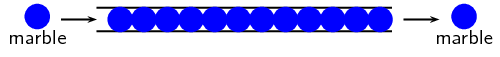If you push one marble into the tube one must come out the other side, if a train locomotive moves all the carriages move immediately because they are connected. This is similar to charges in the wires of a circuit.

The idea is that if a battery started to drive charge in a circuit all the charges start moving instantaneously.

Benjamin Franklin made a guess about the direction of charge flow when rubbing smooth wax with rough wool. He thought that the charges flowed from the wax to the wool (i.e. from positive to negative) which was opposite to the real direction. Due to this, electrons are said to have a negative charge and so objects which Ben Franklin called “negative” (meaning a shortage of charge) really have an excess of electrons. By the time the true direction of electron flow was discovered, the convention of “positive” and “negative” had already been so well accepted in the scientific world that no effort was made to change it.

Copper wire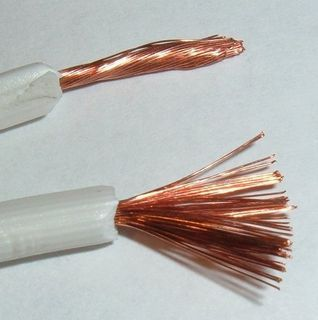Photography on Flickr.com

Current

Current is the rate at which charges moves past a fixed point in a circuit. The units of current are the ampere (A) which is defined as one coulomb per second.

Quantity: Current (I)         Unit name: ampere        Unit symbol: A

Plasma ball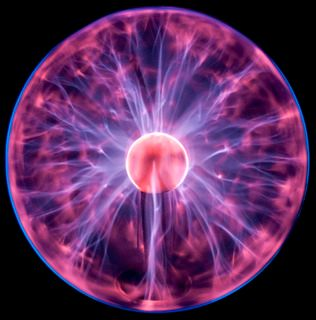Photography by ahisgett on Flickr.com

We use the symbol I to show current and it is measured in amperes (A). One ampere is one coulomb of charge moving in one second ($$\text{C·s^{-1}}$$).

$I = \frac{Q}{\Delta t}$

When current flows in a circuit we show this on a diagram by adding arrows. The arrows show the direction of flow in a circuit. By convention we say that charge flows from the positive terminal on a battery to the negative terminal.

If the voltage is high enough a current can be driven through almost anything. In the plasma ball example on the left, a voltage is created that is high enough to get charge to flow through the gas in the ball. The voltage is very high but the resulting current is very low. This makes it safe to touch.

temp text

### Ammeter (ESAFF)

An ammeter is an instrument used to measure the rate of flow of electric current in a circuit. Since one is interested in measuring the current flowing through a circuit component, the ammeter must be connected in series with the measured circuit component.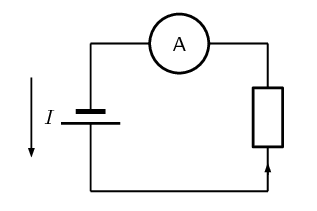Ammeter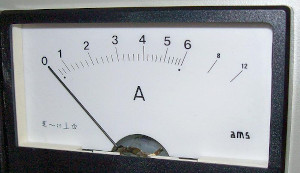## Constructing circuits

Construct circuits to measure the emf and the terminal potential difference for a battery. Some common elements (components) which can be found in electrical circuits include light bulbs, batteries, connecting leads, switches, resistors, voltmeters and ammeters. You have learnt about many of these already. Below is a table with the items and their symbols:

 Component Symbol Usage light bulb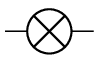glows when charge moves through it battery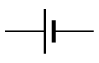provides energy for charge to move switch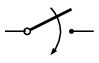allows a circuit to be open or closed resistor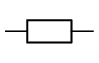resists the flow of charge ORvoltmeter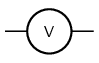measures potential difference ammeter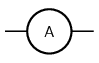measures current in a circuit connecting lead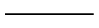connects circuit elements together

Experiment with different combinations of components in the circuits.

The table below summarises the use of each measuring instrument that we discussed and the way it should be connected to a circuit component.

A battery does not produce the same amount of current no matter what is connected to it. While the voltage produced by a battery is constant, the amount of current supplied depends on what is in the circuit.

 Instrument Measured Quantity Proper Connection Voltmeter Voltage In Parallel Ammeter Current In Series

## Using meters

If possible, connect meters in circuits to get used to the use of meters to measure electrical quantities. If the meters have more than one scale, always connect to the first so that the meter will not be damaged by having to measure values that exceed its limits.

## Worked example 1: Calculating current I

An amount of charge equal to $$\text{45}$$ $$\text{C}$$ moves past a point in a circuit in $$\text{1}$$ $$\text{second}$$, what is the current in the circuit?

### Analyse the question

We are given an amount of charge and a time and asked to calculate the current. We know that current is the rate at which charge moves past a fixed point in a circuit so we have all the information we need. We have quantities in the correct units already.

### Apply the principles

We know that:

\begin{align*} I & = \frac{Q}{\Delta t} \\ I & = \frac{\text{45}\text{ C}}{\text{1}\text{ s}} \\ I & = \text{45}\text{ C·s$^{-1}$} \\ I & = \text{45}\text{ A} \end{align*}

### Quote the final result

The current is $$\text{45}$$ $$\text{A}$$.

## Worked example 2: Calculating current II

An amount of charge equal to $$\text{53}$$ $$\text{C}$$ moves past a fixed point in a circuit in $$\text{2}$$ $$\text{s}$$, what is the current in the circuit?

### Analyse the question

We are given an amount of charge and a time and asked to calculate the current. We know that current is the rate at which charge moves past a fixed point in a circuit so we have all the information we need. We have quantities in the correct units already.

### Apply the principles

We know that:

\begin{align*} I & = \frac{Q}{\Delta t} \\ I & = \frac{\text{53}\text{ C}}{\text{2}\text{ s}} \\ I & = \text{26,5}\text{ C·s$^{-1}$} \\ I & = \text{26,5}\text{ A} \end{align*}

### Quote the final result

The current is $$\text{26,5}$$ $$\text{A}$$.

## Worked example 3: Calculating current III

95 electrons move past a fixed point in a circuit in one tenth of a second, what is the current in the circuit?

### Analyse the question

We are given a number of charged particles that move past a fixed point and the time that it takes. We know that current is the rate at which charge moves past a fixed point in a circuit so we have to determine the charge. In the last chapter we learnt that the charge carried by an electron is $$\text{1,6} \times \text{10}^{-\text{19}}$$ $$\text{C}$$.

### Apply the principles: determine the charge

We know that each electron carries a charge of $$\text{1,6} \times \text{10}^{-\text{19}}$$ $$\text{C}$$, therefore the total charge is:

\begin{align*} Q& = 95 \times \text{1,6} \times \text{10}^{-\text{19}}\text{ C} \\ & = \text{1,52} \times \text{10}^{-\text{17}}\text{ C} \end{align*}

### Apply the principles: determine the charge

We know that:

\begin{align*} I & = \frac{Q}{\Delta t} \\ I & = \frac{\text{1,52} \times \text{10}^{-\text{17}}\text{ C}}{\frac{1}{10} \text{ s}} \\ I & = \frac{\text{1,52} \times \text{10}^{-\text{17}}\text{ C}}{1} \times \frac{1}{\frac{1}{10} \text{ s}} \\ I & = \frac{\text{1,52} \times \text{10}^{-\text{17}}\text{ C}}{1}\times \frac{10}{\text{1}\text{ s}} \\ I & = \text{1,52} \times \text{10}^{-\text{16}}\text{ C·s$^{-1}$} \\ I & = \text{1,52} \times \text{10}^{-\text{16}}\text{ A} \end{align*}

### Quote the final result

The current is $$\text{1,52} \times \text{10}^{-\text{16}}$$ $$\text{A}$$.

temp text